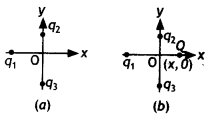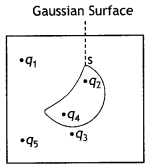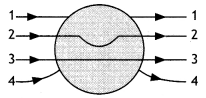# MCQ Questions for Class 12 Physics Chapter 1 Electric Charges and Fields with Answers

Students are advised to practice the NCERT MCQ Questions for Class 12 Physics Chapter 1 Electric Charges and Fields with Answers Pdf free download is available here. MCQ Questions for Class 12 Physics with Answers are prepared as per the Latest Exam Pattern. Students can solve these Electric Charges and Fields Class 12 MCQs Questions with Answers and assess their preparation level.

## Electric Charges and Fields Class 12 MCQs Questions with Answers

Solving the Electric Charges and Fields Multiple Choice Questions of Class 12 Physics Chapter 1 MCQ can be of extreme help as you will be aware of all the concepts. These MCQ Questions on Electric Charges and Fields Class 12 with answers pave for a quick revision of the Chapter thereby helping you to enhance subject knowledge. Have a glance at the MCQ of Chapter 1 Physics Class 12 and cross-check your answers during preparation.

Multiple Choice Questions

Question 1.
In figure (a) two positive charges q2 and q3, fixed along the y-axis, exert a net electric force in the +x direction on a charge q1 fixed along the x-axis. If a positive charge Q is added at (x, 0) shown in figure (b), the force on q1.(a) shall increase along the positive x-axis.
(b) shall decrease along the positive x-axis.
(c) shall point along the negative x-axis.
(d) shall increase but the direction changes because of the intersection of Q with q2 and q3

Question 2.
Suppose the charge of a proton and an electron differ slightly. One of them is -e, the other is (e + Δe). If the net of electrostatic force and gravitational force between two hydrogen atoms placed at a distance d (much greater than atomic size) apart is zero, then Δe is of the order of [Given mass of hydrogen mh = 1.67 × 10-27 kg]
(a) 10-23 C
(b) 10-37 C
(c) 10-47 C
(d) 10-20 C

Question 3.
Consider two concentric spherical metal shells of radii r1 and r2 (r2 > r1). If the outer shell has a charge q and the inner one is grounded, the charge on the inner shell is
(a) $$\frac { -r_2q }{r_1}$$
(b) zero
(c) $$\frac { -r_1q }{r_2}$$
(d) -q

Question 4.
Four charges equal to -Q are placed at the four corners of a square and a charge q is at its centre. If the system is in equilibrium the value of q is
(a) $$\frac { -Q }{4}$$ (1 + 2√2)
(b) $$\frac { Q }{4}$$ (1 + 2√2)
(c) $$\frac { -Q }{2}$$ (1 + 2√2)
(d) $$\frac { Q }{2}$$ (1 + 2√2)

Question 5.
A point positive charge is brought near an isolated conducting sphere. The electric field is best given by(a) figure (i)
(b) figure (ii)
(c) figure (iii)
(d) figure (iv)

Question 6.
Five charges q1, q2, q3, q4 and q are fixed at their positions as shown in figure S is a Gaussian surface. The Gauss’s law is given by $$\oint_{s}$$ $$\vec{E} \cdot \overrightarrow{d s}$$ = $$\frac { q }{ε_0}$$Which of the following statements is, correct?
(a) E on the LHS of the above equation will have a contribution from q1, q5 and q3 while q on the RHS will have a contribution from q2 and q4 only.
(b) E on the LHS of the above equation will have a contribution from all charges while q on the RHS will have a contribution from q2 and q4 only.
(c) E on the LHS of the above equation will have a contribution from all charges while q on the RHS will have a contribution from q1, q3 and q5 only.
(d) Both E on the LHS and q on the RHS will have contributions from q2 and q4 only.

Question 7.
A long cylindrical shell carries positive surface charge σ in the upper half and negative surface charge -σ in the lower half. The electric field lines around the cylinder will look like figure given in: (figure are schematic and not drawn to scale)Question 8.
Three infinitely charged sheets are kept parallel to X-Y plane having charge densities as shown in figure. Then, the value of electric field at P is(a) $$\frac { -4σ }{ε_0}$$
(b) $$\frac { σ }{ε_0}$$
(c) $$\frac { -2σ }{ε_0}$$
(d) $$\frac { 2σ }{ε_0}$$

Question 9.
Which one of the following graphs represents the variation of electric field strength E with distance r from the centre of a uniformly charged non-conducting sphere?Question 10.
A metallic solid sphere is placed in a uniform electric field. The lines of force follow the path (s) shown in the figure as(a) 1
(b) 2
(c) 3
(d) 4

Fill in the Blanks

Question 1.
The possessing of charge by a body, which is an integral multiple of some smallest charge, is called ……………. of charge.

Question 2.
Two unequal charges exert …………… magnitude force on each other.

Question 3.
If a silk cloth rubbed with a glass rod has a charge q = -1.6 × 10-19 C, then the charge on the glass rod will be …………..

Question 4.
The net charge in a system remains conserved. This is called ………….. of charge.

Question 5.
…………. is a simple apparatus to detect charge on a body.

Question 6.
Those substances which do not allow electricity to pass through them easily are called …………..

Question 7.
A process in which a body is charged by another body without coming in contact is called …………

Question 8.

Question 9.
…………. due to a charge Q at a point in space may be defined as the force that a unit positive charge would experience if placed at that point.

Question 10.
Two point charges are separated by some distance inside vacuum. When space between the charges is filled by some dielectric, the force between two point charges …………..

Question 11.
The SI unit of electric field is …………….

Answer: N C-1 or V m-1

Question 12.
Electric field lines never …………. each other.

Question 13.
Electric dipole moment is a ………….. quantity.

Question 14.
The torque acting on an electric dipole will tend to align the dipole ……………. with the field E.

Question 15.
An electric dipole is placed inside a uniform electric field. When it is rotated from unstable equilibrium to stable equilibrium in a uniform electric field, its potential energy ……………

Question 16.
Direction of electric field intensity due to a dipole on equatorial point is ……………. to the direction of dipole moment.

Question 17.
A charge Q. is enclosed by a Gaussian spherical surface of radius R. If the radius is doubled, then the electric ……………. will remain same.

Question 18.
The electric flux through a region enclosing a charge q is ………….

Question 19.
Electric flux is a ………… quantity.

Question 20.
Gauss’s law is true for any ………….. surface, no matter what its shape or size is.

Question 21.
Gauss’s law is often useful towards a much easier calculation of the electrostatic field when the system has some …………

Question 22.
Net electric flux from a closed surface does not depend upon distribution of ……………. inside the surface.

Question 23.
The value of electric field inside a hollow shell is ……………

Question 24.
Net electrostatic field inside a positively charged conductor is …………..

Question 25.
Inside a conductor, electrostatic field is ……………